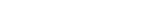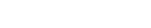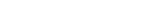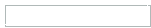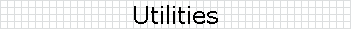In addition to our main analysis programs FEMRDYN and iSTRDYN, we also offer two utility programs:

BAFLER (BAlancing of FLExible Rotors):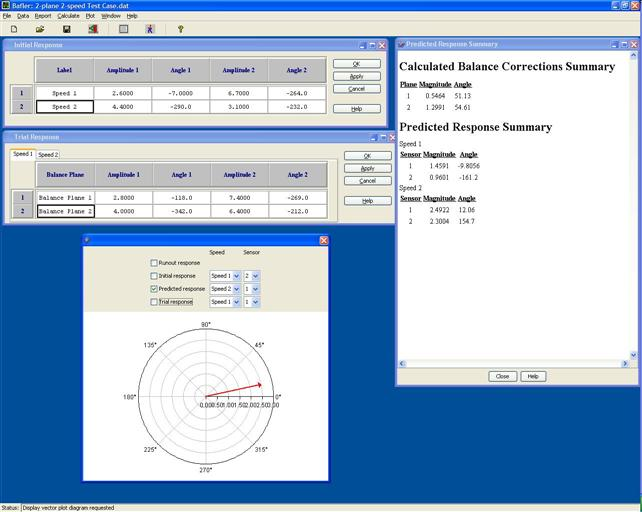For balancing a multi-plane rotor at several speeds, BAFLER determines correction values using a least-squares weighting factor approach.  Program features include: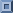Multiple correction planes, with provision for handling initial weightsMultiple sensor planes, with capability to subtract initial runout valuesMultiple balance speedsTabular input of all balancing dataComplete listings of all input and calculated quantitiesGraphical display of response and corrections: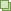Runout (if any) at each sensorInitial response at each speed and sensorTrial response at each speed and sensorPredicted response at each speed and sensorInitial weights (if any) at each correction planeCalculated weights at each correction planeExport of all listings and plotted quantitiesAll input quantities saved to XML filePlatform independent (Windows, Unix, Linux, Mac)Integration Routines:

As a service to the engineering community, we are making available two different Java programs that integrate a system of first order differential equations. We use the algorithms in our rotor dynamics codes.  The programs are contained in a WinZip file, with the following contents: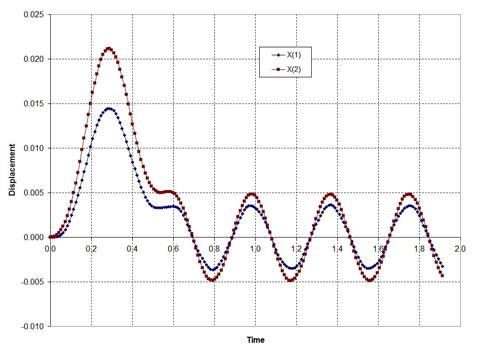A Java source file with the integration done entirely with double arraysA Java source file with the integration performed using JAMA (Java Matrix Algebra)An Excel spreadsheet that plots the displacements (as shown in figure)A text file that lists the results from using doubles and JAMA

Both source files integrate a 2 DOF spring-mass problem by converting the 2nd order system to first order.  You may use these codes free of charge, as long as you agree to the following:

1. You give credit to DynaTech Engineering as the original author.

2. You assume all liability for use of the software.